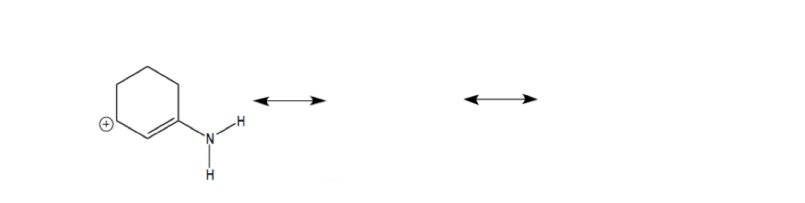# Problem: A Lewis formula is shown on the left below. Analyze the structure and determine where you need the electron arrow(s) to generate the proper electron flow to its resonance contributors. Draw the electron arrows for each step, the resulting structures, and circle the major contributor.

###### Problem Details

A Lewis formula is shown on the left below. Analyze the structure and determine where you need the electron arrow(s) to generate the proper electron flow to its resonance contributors. Draw the electron arrows for each step, the resulting structures, and circle the major contributor.